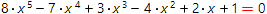# Solve polynomial equations onlinePolynomial equation of n-th degree has the form:

a∙x n + b∙x n− 1 + c∙x 2 + d∙x + e = 0

There are no formulas to find the roots of polynomial equations of n-th degree (n ≥ 5) by its coefficients. It was for the first time proved by the Norway mathematician Niels Abel.

However, the roots of the n-th degree polynomial can be found with any required accuracy by using the numerical methods. Our calculator uses the Laguerre numerical method.

To begin the calculations, the required accuracy and the maximum number of iterations to spend must be specified.

Polynomial equations solverInput polynomial equation: